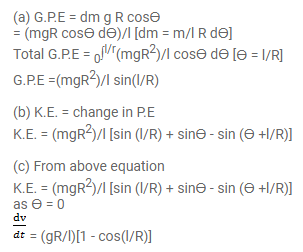Deepak Scored 45->99%ile with Bounce Back Crack Course. You can do it too!

# A chain of length 1 and mass m lies on the surface of a smooth

Question:

A chain of length 1 and mass $m$ lies on the surface of a smooth sphere of radius $R>1$ with one end tied to the top of the sphere.

(a) Find the gravitational potential energy of the chain with reference level at the centre of the sphere.

(b) Suppose the chain is released and slides down the sphere. Find the kinetic energy of the chain, when it has slid through an angle 9 .

(c) Find the tangential acceleration $\mathrm{dv} / \mathrm{dt}$ of the chain when the chain starts sliding down.

Solution: